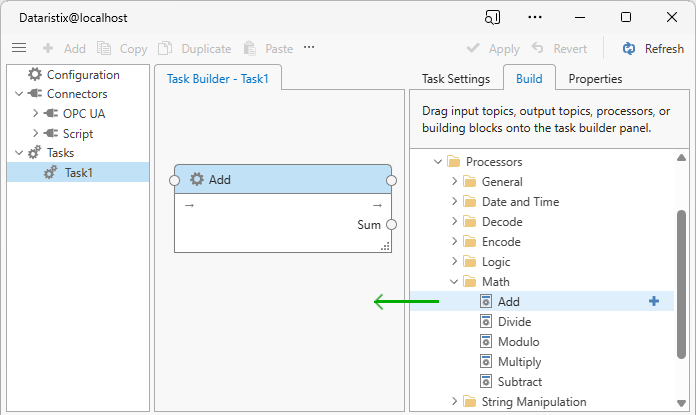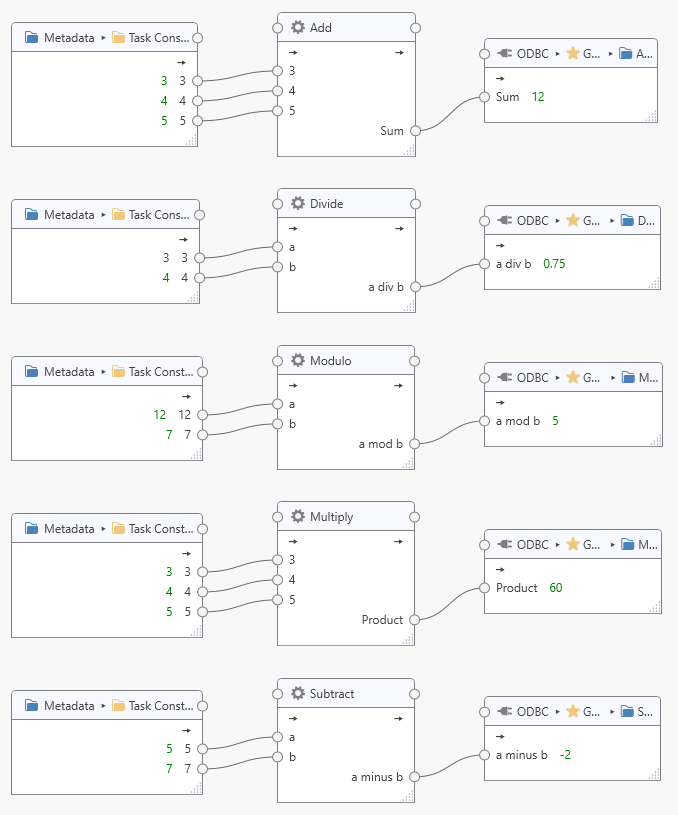# Math Processors¶

Math processors are installed with the Dataristix core application. These processors perform a mathematical operation on input values and return a numerical result.

Available mathematical operations are:

Calculates the sum of all input values.
• Divide
Divides one number by the other and returns the result.
• Modulo
Calculates the remainder after division of two numbers.
• Multiply
Calculates the product of all input values.
• Subtract
Caclulates the difference of two numbers.

The processors attempt to convert any input value to a numerical type before peforming the calculations.

If an input value is a one-dimensional array then the Add or Multiply operation is performed for each element. Array values are invalid inputs for other operations.

If any input value is of “uncertain” quality then the output value will also be of uncertain quality.

If any input value is of “bad” quality then the output value will also be of bad quality.## Configuration¶

Select the processor item in the “Task Builder” panel to view its properties.You can change the following properties for each of the mathematical processors:

• Topic
The title of the processor as it appears within the task builder.

Press the “Apply” button to commit your changes.

## Example¶

The following example shows a task using various inputs and the results after processing by the mathematical processors.